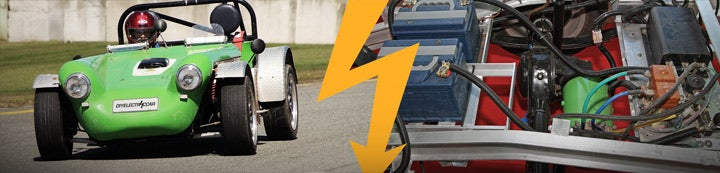1 - 3 of 3 Posts

#### EVDL List

·
##### Registered
Joined
·
70 Posts
Discussion Starter · ·
I don't know the formula for figuring my mpg
equivalent for my conversion.
My nominal pack voltage is 156volts and I'm drawing
right around 90amps on level ground at 60mph(234
wh/mi). Electricity costs me 11.5 cents per kilowatt.
If you could share the formula so I can save it for
future testing this would really help.
Thanks,
Jeff McCabe

#### EVDL List

·
##### Registered
Joined
·
70 Posts
Discussion Starter · ·
If you have a Emeter, than you can use the watts per mile instead of the AH.
Of course this will be the average amount of battery amperes times battery
voltages.

Or if you have a AC input volt-amp meter and calculated the volts x amps =
watts for a constant current charger and average out the voltage curve or
better yet, use a AC watt meter to give you how many kilowatt hr it takes to
charge the batteries for a given number of miles.

Or just take the average battery amperes times the average voltages for a
ball park estimate for the wattages.

Now lets say you use about 10k watts to go 50 miles, then if you paying 11.5
cents per kilowatt, then the 50 miles will cost you about \$0.115 x 10 =
\$1.15

If gasoline is \$3.45 a gallon, then \$3.45/\$1.15 = 3

So you can go 3 times the distance with the electric with \$3.45 or would be
equivalent to 3 x 50 miles = 150 miles per gallon equiavlent or MPGE

The formula now becomes:

MPGE = ((cost of gal of gas)/(kw use x cost per kw))x miles travel

Roland

----- Original Message -----
From: "Jeff Mccabe" <[email protected]>
To: "ev-list" <[email protected]>
Sent: Wednesday, August 01, 2007 3:26 PM
Subject: MPG Equivalent ?

> I don't know the formula for figuring my mpg
> equivalent for my conversion.
> My nominal pack voltage is 156volts and I'm drawing
> right around 90amps on level ground at 60mph(234
> wh/mi). Electricity costs me 11.5 cents per kilowatt.
> If you could share the formula so I can save it for
> future testing this would really help.
> Thanks,
> Jeff McCabe
>
>

#### EVDL List

·
##### Registered
Joined
·
70 Posts
Discussion Starter · ·
Roland,

--- Roland Wiench <[email protected]> wrote:

> If you have a Emeter, than you can use the watts per
> mile instead of the AH.
> Of course this will be the average amount of battery
> amperes times battery
> voltages.
>
> Or if you have a AC input volt-amp meter and
> calculated the volts x amps =

My conversion is a DC system?.
http://www.austinev.org/evalbum/736.
This is how I came up with the watts: 156v x 90amp
@60mph.= 14040 wh./ 60mph=234wh/mi. is that correct ?

> watts for a constant current charger and average out
> the voltage curve or
> better yet, use a AC watt meter to give you how many
> kilowatt hr it takes to
> charge the batteries for a given number of miles.
>
> Or just take the average battery amperes times the
> average voltages for a
> ball park estimate for the wattages.
>
> Now lets say you use about 10k watts to go 50 miles,
> then if you paying 11.5
> cents per kilowatt, then the 50 miles will cost you
> about \$0.115 x 10 =
> \$1.15
>
> If gasoline is \$3.45 a gallon, then \$3.45/\$1.15 = 3
>
> So you can go 3 times the distance with the electric
> with \$3.45 or would be
> equivalent to 3 x 50 miles = 150 miles per gallon
> equiavlent or MPGE
>
> The formula now becomes:
>
> MPGE = ((cost of gal of gas)/(kw use x cost per
> kw))x miles travel

Thanks, I can follow this "mpge"
What I want to do is just find out my mpge for
several speeds, say 25mph, 35 and 60mph. Just to get
an idea of my efficiency.
Thanks again,
Jeff

>
> Roland
>
> ----- Original Message -----
> From: "Jeff Mccabe" <[email protected]>
> To: "ev-list" <[email protected]>
> Sent: Wednesday, August 01, 2007 3:26 PM
> Subject: MPG Equivalent ?
>
>
> > I don't know the formula for figuring my mpg
> > equivalent for my conversion.
> > My nominal pack voltage is 156volts and I'm
> drawing
> > right around 90amps on level ground at 60mph(234
> > wh/mi). Electricity costs me 11.5 cents per
> kilowatt.
> > If you could share the formula so I can save it
> for
> > future testing this would really help.
> > Thanks,
> > Jeff McCabe
> >
> >
>
>

1 - 3 of 3 Posts